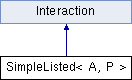ParM  parm A molecular dynamics library
SimpleListed< A, P > Class Template Reference

#include <interaction.hpp>

Inheritance diagram for SimpleListed< A, P >:Public Member Functions

SimpleListed ()

flt energy (Box &box)
Potential energy due to this Interaction. More...

flt pressure (Box &box)
Partial pressure due to this Interaction. More...

uint size ()

void set_forces (Box &box)

flt set_forces_get_pressure (Box &box)
Set forces (Atom.f) and return $$P = \sum_{\left<i,j \right>} \vec r_{ij} \cdot \vec F_{ij}$$ at the same time (see pressure()). More...

vector< A > & atom_list ()

~SimpleListed ()Public Member Functions inherited from Interaction
virtual Matrix stress (Box &box)
The force-moment tensor for the current simulation: More...

virtual ~Interaction ()

Protected Attributes

vector< A > atoms

Constructor & Destructor Documentation

template<class A , class P >
 SimpleListed< A, P >::SimpleListed ( )
inline
template<class A , class P >
 SimpleListed< A, P >::~SimpleListed ( )
inline

Member Function Documentation

template<class A , class P >
 void SimpleListed< A, P >::add ( A atm )
inline
template<class A , class P >
 vector& SimpleListed< A, P >::atom_list ( )
inline
template<class A , class P >
 flt SimpleListed< A, P >::energy ( Box & box )
virtual

Potential energy due to this Interaction.

Implements Interaction.

template<class A , class P >
 flt SimpleListed< A, P >::pressure ( Box & box )
virtual

Partial pressure due to this Interaction.

$$P = \sum_{\left<i,j \right>} \vec r_{ij} \cdot \vec F_{ij}$$, or equivalently $$P = \sum_i \vec r_i \cdot \vec F_i$$

Note that the full pressure involves all interactions and temperature, and needs to be normalized by $$\frac{1}{dV}$$ where $$d$$ is the number of dimensions and $$V$$ is the volume.

Implements Interaction.

template<class A , class P >
 void SimpleListed< A, P >::set_forces ( Box & box )
virtual

Implements Interaction.

template<class A , class P >
 flt SimpleListed< A, P >::set_forces_get_pressure ( Box & box )
virtual

Set forces (Atom.f) and return $$P = \sum_{\left<i,j \right>} \vec r_{ij} \cdot \vec F_{ij}$$ at the same time (see pressure()).

Reimplemented from Interaction.

template<class A , class P >
 uint SimpleListed< A, P >::size ( )
inline

Member Data Documentation

template<class A , class P >
 vector SimpleListed< A, P >::atoms
protected

The documentation for this class was generated from the following file: